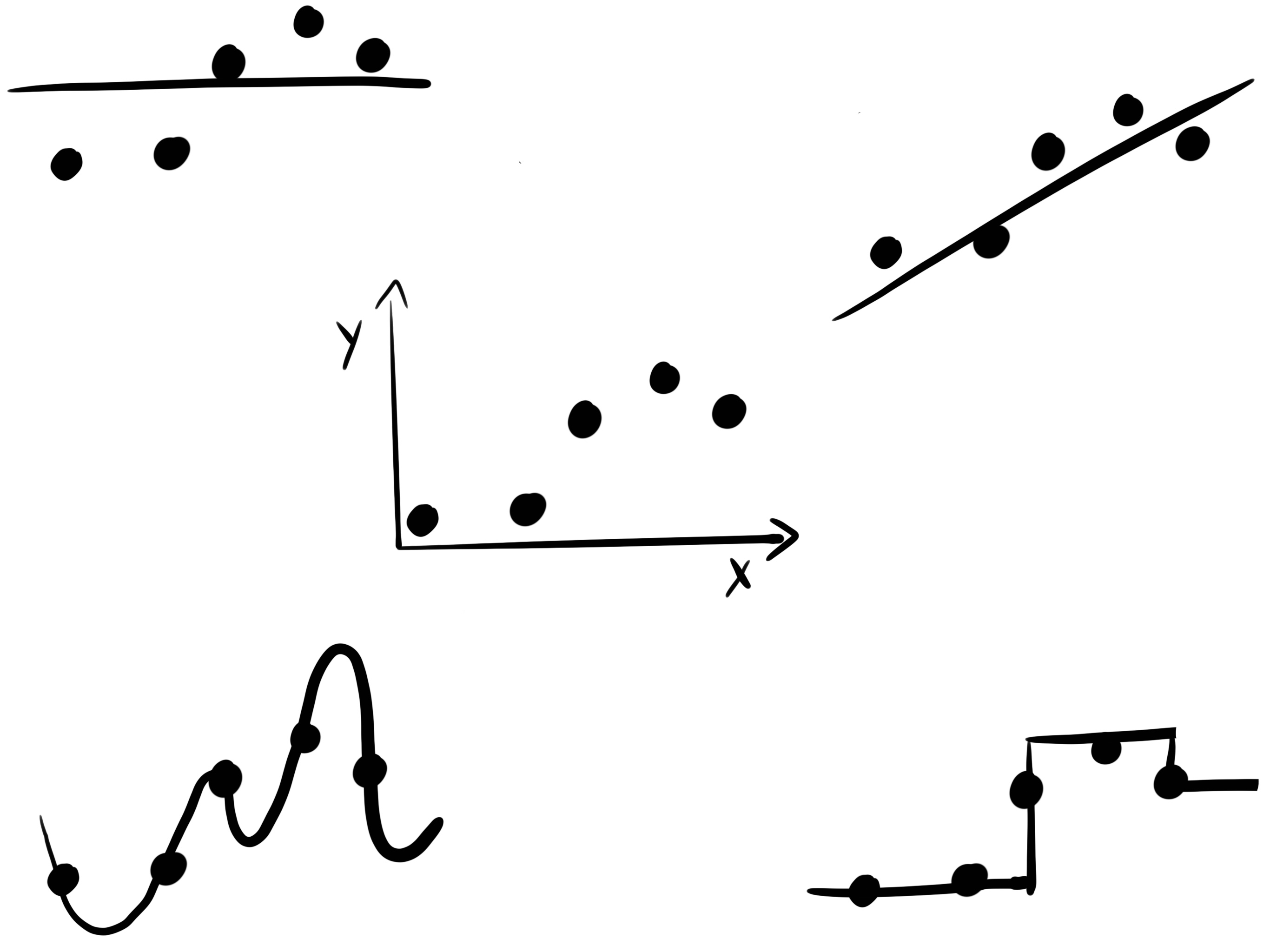# Inductive Bias

A machine learning model has to learn general rules from seeing examples. Learning something general from something specific is called induction (the opposite is called deduction which would be to go from general law to specific conclusions). A bias means preferring one solution over another. Together, inductive bias means “preferring a solution over another after seeing some examples”. That’s what machine learning models do. And each model has their individual biases. Because each type of model solves the problem of learning some generalization from individual examples in a different way.

We want the ML model to be useful beyond the training data. If you want to have a model that only works for the training data, you need a database.

Look at the following image. We have some points with an x value and a y value, and we want a good curve that helps us predict the y values from x. There are many (infinitely) many curves that can be used and that might yield a good prediction. But which way should the curve look like? To some degree the model learns how the function should look like, based on the data examples. But especially where we don‘t have much data or where we get outside the range of x, the model has to work with assumptions. The image shows an example of a linear curve, a flat curve, a step function or some more complex function.Examples of inductive bias:

• The linear model assumes that the target has a linear relationship with each of the input features
• Decision trees learn constant models in their nodes.
• A convolutional neural network with its composition of layers imposes a bias of hierarchical processing.
• Bayesian modeling: The bias here is expressed a lot through the choice of priors (which tells the model what happens when not much data is available).

Inductive biases come not only from the choices of a specific model, but from every choice in the modeling process. Choosing the L1 loss instead of the L2 loss favors sparse solutions. Representing a categorical feature as a single feature with numbers instead of one-hot-encoding gives an order to the features. And if that order is meaningful, it gets simpler for the model to learn. Models with different inductive biases can still have very similar performance on test data. This multiplicity of models are called Rashomon Sets.